# Loop space of a based topological space

## Definition

### As a (based) topological space

Suppose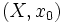$(X,x_0)$ is a based topological space, i.e.,$X$ is a topological space and$x_0$ is a point in$X$. The loop space of$(X,x_0)$, denoted$\Omega(X,x_0)$, is defined as follows:

• As a set, it is the set of all continuous maps from the based unit circle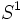$S^1$ (i.e., the unit circle with a fixed basepoint) to$(X,x_0)$. In other words, all these maps send the chosen basepoint of$S^1$ to$x_0$.
• The topology on this set is the compact-open topology (or rather, the subspace topology from the compact-open topology on all continuous maps from$S^1$ to$X$).
• The basepoint of this topological space is chosen as the constant loop that stays at the point$x_0$.

## As a H-space=

For convenience, we treat the unit circle as the quotient of the closed unit interval$[0,1]$ under the identification of$0$ and$1$, and the identified$0 \sim 1$ point is treated as the basepoint.

The loop space$\Omega(X,x_0)$ admits a multiplicative structure by concatenation and reparametrization, where, for loops$f_1$ and$f_2$, we define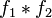$f_1 *f_2$ as the loop:$t \mapsto \lbrace \begin{array}{rl} f_1(2t), & 0 \le t < 1/2 \\ f_2(2t - 1), & 1/2 \le t \le 1 \\\end{array}$

This multiplicative structure is continuous, making the loop space a topological magma. However, it is not a topological monoid, because the multiplication is not strictly associative and does not have a strict identity element. Instead, it is a H-space, in the sense that the multiplication is associative up to homotopy and there is an element that works as an identity element up to homotopy:

Condition Proof
Associativity up to homotopy homotopy between composites associated in different ways
Identity element up to homotopy homotopy between loop and composite with constant loop

What is important is not just that there exist individual homotopies for the associativity of each triple, but that these homotopies vary continuously, so that we get a homotopy at the level of the topological space$\Omega(X,x_0)$.

## Relation with other constructs

### Relationship with fundamental group

The fundamental group of a based topological space$(X,x_0)$, denoted$\pi_1(X,x_0)$, can be identified with the set of path components of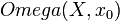$Omega(X,x_0)$. In other words: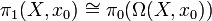$\! \pi_1(X,x_0) \cong \pi_0(\Omega(X,x_0))$

The identification is as follows: we know that the elements of$\pi_1(X,x_0)$ are precisely the homotopy classes of loops in$X$ based at$x_0$. The homotopy classes, in turn, are precisely the path components of$\Omega(X,x_0)$, because a homotopy of based loops is a path in the space of based loops under the compact-open topology.

In addition to the identification as a set, we can also make the identification as a group. The left side has a group structure under concatenation. On the right side, the H-space structure of$\Omega(X,x_0)$ induces a monoid structure on the space$\pi_0(\Omega(X,x_0))$ of its path components. That monoid turns out to be a group, and the identification is a group isomorphism.

### Relationship with higher homotopy groups

More generally, we have the following relationship:$\! \pi_k(X,x_0) \cong \pi_{k-1}(\Omega(X,x_0))$

In fact, for$k \ge 2$, both sides are naturally abelian groups, and the natural identification is an isomorphism of abelian groups.

### Iterated loop spaces

We can also consider the iterated loop space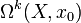$\Omega^k(X,x_0)$. This is obtained by iterating the loop space construction$k$ times. Note that at each stage, the new basepoint is chosen as the constant loop taking the old basepoint as its value.